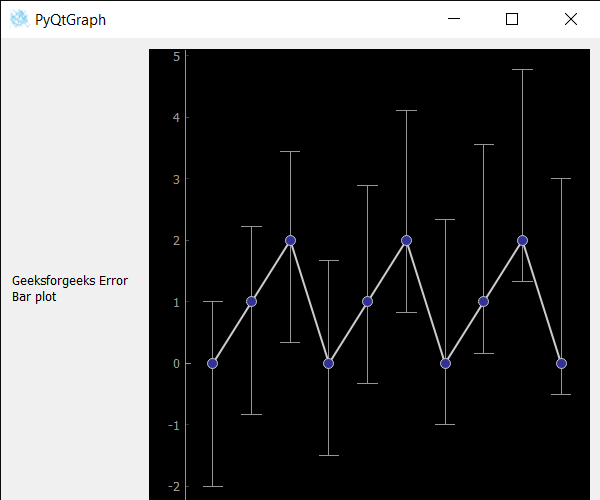GeeksforGeeks App
Open AppBrowser
Continue

# PyQtGraph – Error Bar Graph

In this article we will see how we can create a error bar graph in the PyQtGraph module. PyQtGraph is a graphics and user interface library for Python that provides functionality commonly required in designing and science applications. Its primary goals are to provide fast, interactive graphics for displaying data (plots, video, etc.). Error bars are graphical representations of the variability of data and used on graphs to indicate the error or uncertainty in a reported measurement. They give a general idea of how precise a measurement is, or conversely, how far from the reported value the true value might be.

We can create a plot window and create error bar graph on it with the help of commands given below.

```# creating a pyqtgraph plot window
plt = pg.plot()

# creating a error bar item object
error = pg.ErrorBarItem(x=x, y=y, top=top, bottom=bottom, beam=0.5)```

In order to create a error bar graph in pyqtgraph we have to do the following
1. Import the pyqtgraph module
2. import other modules as well like numpy and pyqt5
3. Create a main window class
4. Create a plot window and error bar item
5. Set x-axis, y-axis and upper and lower error values to the error bar item
6. Create a grid layout
7. Add error bar item to the plot window and plot the data on plot window
9. Set layout widget as the central widget

Below is the implementation

## Python3

 `# importing Qt widgets``from` `PyQt5.QtWidgets ``import` `*` `# importing system``import` `sys` `# importing numpy as np``import` `numpy as np` `# importing pyqtgraph as pg``import` `pyqtgraph as pg``from` `PyQt5.QtGui ``import` `*``from` `PyQt5.QtCore ``import` `*` `from` `collections ``import` `namedtuple` `class` `Window(QMainWindow):` `    ``def` `__init__(``self``):``        ``super``().__init__()` `        ``# setting title``        ``self``.setWindowTitle(``"PyQtGraph"``)` `        ``# setting geometry``        ``self``.setGeometry(``100``, ``100``, ``600``, ``500``)` `        ``# icon``        ``icon ``=` `QIcon(``"skin.png"``)` `        ``# setting icon to the window``        ``self``.setWindowIcon(icon)` `        ``# calling method``        ``self``.UiComponents()` `        ``# showing all the widgets``        ``self``.show()` `    ``# method for components``    ``def` `UiComponents(``self``):` `        ``# creating a widget object``        ``widget ``=` `QWidget()` `        ``# creating a label``        ``label ``=` `QLabel(``"Geeksforgeeks Error Bar plot"``)` `        ``# making label do word wrap``        ``label.setWordWrap(``True``)` `        ``# setting configuration options``        ``pg.setConfigOptions(antialias``=``True``)` `        ``# creating x-axis values``        ``x ``=` `np.arange(``10``)` `        ``# creating y-axis values``        ``y ``=` `np.arange(``10``) ``%` `3` `        ``# creating upper bound values``        ``top ``=` `np.linspace(``1.0``, ``3.0``, ``10``)` `        ``# creating lower bound values``        ``bottom ``=` `np.linspace(``2``, ``0.5``, ``10``)` `        ``# creating a plot window``        ``plt ``=` `pg.plot()` `        ``# creating a error bar item``        ``error ``=` `pg.ErrorBarItem(x``=``x, y``=``y, top``=``top, bottom``=``bottom, beam``=``0.5``)` `        ``# adding error bar item to the plot window``        ``plt.addItem(error)` `        ``# plotting the data on plot window``        ``plt.plot(x, y, symbol``=``'o'``, pen``=``{``'color'``: ``0.8``, ``'width'``: ``2``})` `        ``# Creating a grid layout``        ``layout ``=` `QGridLayout()` `        ``# minimum width value of the label``        ``label.setMinimumWidth(``130``)` `        ``# setting this layout to the widget``        ``widget.setLayout(layout)` `        ``# adding label in the layout``        ``layout.addWidget(label, ``1``, ``0``)` `        ``# plot window goes on right side, spanning 3 rows``        ``layout.addWidget(plt, ``0``, ``1``, ``3``, ``1``)` `        ``# setting this widget as central widget of the main window``        ``self``.setCentralWidget(widget)` `# create pyqt5 app``App ``=` `QApplication(sys.argv)` `# create the instance of our Window``window ``=` `Window()` `# start the app``sys.exit(App.``exec``())`

Output :My Personal Notes arrow_drop_up